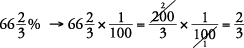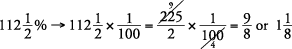## Changing Percents, Decimals, and Fractions

A fraction whose denominator is 100 is called a percent. The word percent means hundredths (per hundred).

Using the step‐by‐step methods of changing decimals, percents, and fractions makes the conversions straightforward and simple to complete.

To change decimals to percents,

Move the decimal point two places to the right.

Insert a percent sign.

Change to percents.

0.55

0.02

3.21

0.004

0.55 = 55%

0.02 = 2%

3.21 = 321%

0.004 = 0.4%

To change percents to decimals,

Eliminate the percent sign.

Move the decimal point two places to the left. (Sometimes, adding zeros is necessary.)

Change to decimals.

24%

7%

250%

24% = 0.24

7% = 0.07

250% = 2.50 = 2.5

To change fractions to percents,

Change to a decimal.

Change the decimal to a percent.

Change to percents.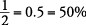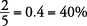There are two simple methods for changing percents to fractions.

Drop the percent sign.

Write over one hundred.

Simplify if possible.

4a

(Applying method 1) Change to fractions.

13%

70%

45%

130%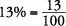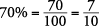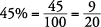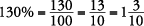Drop the percent sign.

Multiply by.

Simplify if possible.

4b

(Applying method 2) Change to fractions.